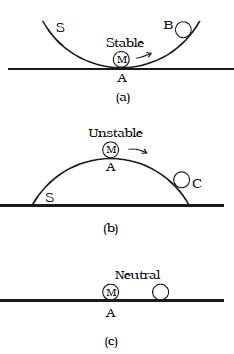# Explain Equilibrium of Bodies

If a marble M is placed on a curved surface of a bowl S. it rolls down and settles in equilibrium at the lowest point A (Fig. a). This equilibrium position corresponds to minimum potential energy. If the marble is disturbed and displaced to a point B. its energy increases When it is released, the marble rolls back to A. Thus the marble at the position A is said to be in stable equilibrium Suppose now that the bowl S is inverted and the marble is placed at its top point, at A (Fig. b).

If the marble is displaced slightly to the point C, its potential energy is lowered and tends to move further away from the equilibrium position to one of lowest energy. Thus the marble is said to be in unstable equilibrium.Fig: Equilibrium of rigid bodies

Suppose now that the marble is placed on a plane surface (Fig. c). If it is displaced slightly, its potential energy does not change. Here the marble is said to be in neutral equilibrium. Equilibrium is thus stable, unstable or neutral according to whether the potential energy is minimum, maximum or constant.

We may also characterize the stability of a mechanical system by noting that when the system is disturbed from its position of equilibrium, the forces acting on the system may

• Tend to bring back to its original position if potential energy is a minimum, corresponding to the stable equilibrium.
• Tend to move it farther away if potential energy is maximum, corresponding unstable equilibrium.
• Tend to move either way if potential energy is a constant corresponding to neutral equilibrium.